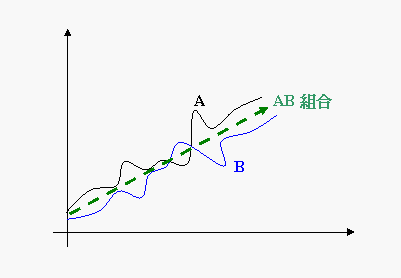## 平均報酬不會相互抵銷## 美國股票及債券長期之相關係數為0.2## 附註：兩組資產配置之數學

 平均報酬率 (μ) 標準差 (σ) 資產比例 (w) A資產(股票型基金) μa = 14% σa = 17% wa = 0.5 B資產(債券型基金) μb = 8% σb = 6% wb = 0.5 AB資產相關係數 ρab = 0.2

### 相配置後：

Excel 表示 = ((0.5*17%)^2+(0.5*6%)^2+2*0.2*0.5*17%*0.5*6%)^0.5 = 9.6%

= ((0.5*17%)^2+(0.5*6%)^2-2*0.2*0.5*17%*0.5*6%)^0.5 = 8.4%